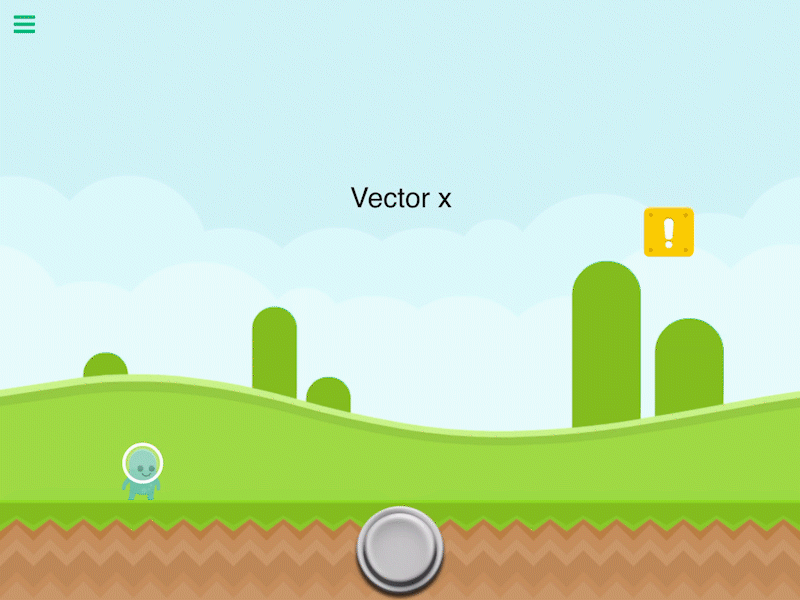# Calculate Direction

Description

Calculates the vector between 2 objects. The vector goes from Object A to Object B. The magnitude of the vector will be different depending on each zoom. So if you zoom out the magnitude will be less because it visually looks like there is less distance between them. To compensate for this, you must multiply by the zoom of your screen.

Properties

Triggers Immediately

 Object A By default this is the current object selected. You can change this to any other object in your scene. Object B Another object in your scene to calculate the vector between. Anchor Use the anchor to offset the position. By default the vector is calculated between the center of 2 objects.

Outputs

 Vector X Outputs the direction on the X-axis as a vector value. Vector Y Outputs the direction on the Y-axis as a vector value. Angle Outputs the angle between the two objects.

Related

Calculate Distance

Get Bounding Box

Get Time

Is IntersectingWas this article helpful?
0 out of 0 found this helpful
Have more questions? Submit a request

#### 0 Comments

Please sign in to leave a comment.